Next: 7.2 Shortest Paths Problem Up: 7.1 Directed Graphs Previous: 7.1 Directed Graphs

## 7.1.1 Data Structures for Graph Representation

1.
Adjacency Matrix: The matrix is of order (n x n) where n is the number of vertices. The adjacency matrix for the graph in Figure 7.2 is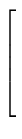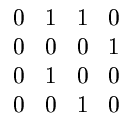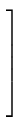2.
Adjacency List: Figures 7.2 and 7.3 provide two different adjacency list representations for the graph of Figure 7.1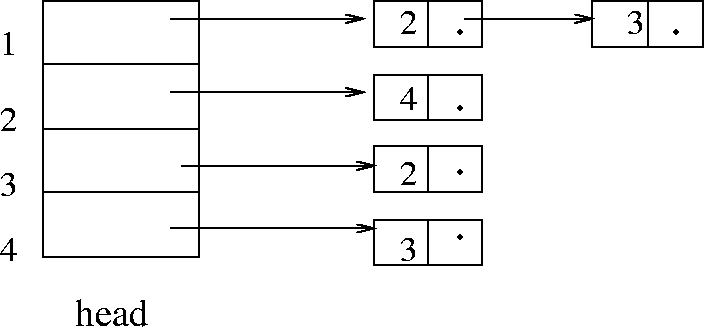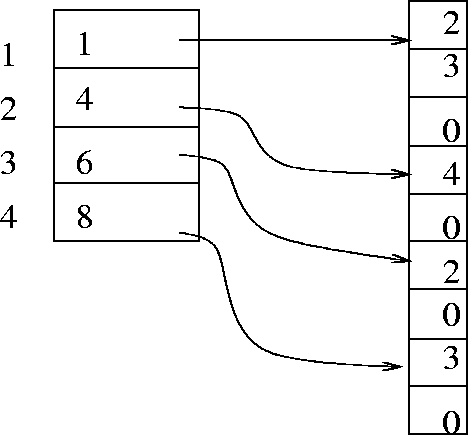eEL,CSA_Dept,IISc,Bangalore